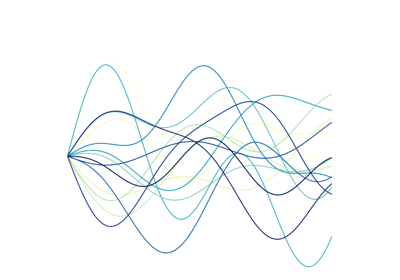# tslearn.preprocessing.TimeSeriesScalerMeanVariance¶

class tslearn.preprocessing.TimeSeriesScalerMeanVariance(mu=0.0, std=1.0)[source]

Scaler for time series. Scales time series so that their mean (resp. standard deviation) in each dimension is mu (resp. std).

Parameters: mu : float (default: 0.) Mean of the output time series. std : float (default: 1.) Standard deviation of the output time series.

Notes

This method requires a dataset of equal-sized time series.

NaNs within a time series are ignored when calculating mu and std.

Examples

>>> TimeSeriesScalerMeanVariance(mu=0.,
...                              std=1.).fit_transform([[0, 3, 6]])
array([[[-1.22474487],
[ 0.        ],
[ 1.22474487]]])
>>> TimeSeriesScalerMeanVariance(mu=0.,
...                              std=1.).fit_transform([[numpy.nan, 3, 6]])
array([[[nan],
[-1.],
[ 1.]]])


Methods

 fit(X[, y]) A dummy method such that it complies to the sklearn requirements. fit_transform(X[, y]) Fit to data, then transform it. get_params([deep]) Get parameters for this estimator. set_params(**params) Set the parameters of this estimator. transform(X[, y]) Fit to data, then transform it.
fit(X, y=None, **kwargs)[source]

A dummy method such that it complies to the sklearn requirements. Since this method is completely stateless, it just returns itself.

Parameters: X Ignored self
fit_transform(X, y=None, **kwargs)[source]

Fit to data, then transform it.

Parameters: X : array-like of shape (n_ts, sz, d) Time series dataset to be rescaled. numpy.ndarray Resampled time series dataset.
get_params(deep=True)[source]

Get parameters for this estimator.

Parameters: deep : bool, default=True If True, will return the parameters for this estimator and contained subobjects that are estimators. params : dict Parameter names mapped to their values.
set_params(**params)[source]

Set the parameters of this estimator.

The method works on simple estimators as well as on nested objects (such as Pipeline). The latter have parameters of the form <component>__<parameter> so that it’s possible to update each component of a nested object.

Parameters: **params : dict Estimator parameters. self : estimator instance Estimator instance.
transform(X, y=None, **kwargs)[source]

Fit to data, then transform it.

Parameters: X : array-like of shape (n_ts, sz, d) Time series dataset to be rescaled numpy.ndarray Rescaled time series dataset

## Examples using tslearn.preprocessing.TimeSeriesScalerMeanVariance¶Longest Common SubsequenceLB_KeoghsDTW multi path matchingLongest Commom Subsequence with a custom distance metricDTW computation with a custom distance metric1-NN with SAX + MINDISTKShapeKernel k-meansk-meansEarly ClassificationModel PersistencePAA and SAX featuresDistance and Matrix Profiles